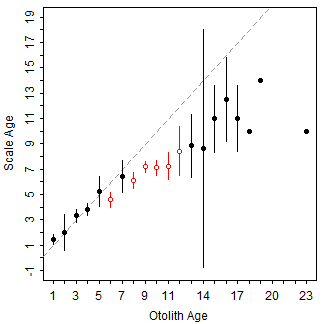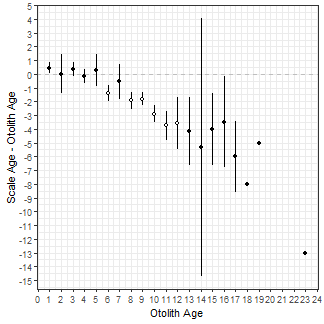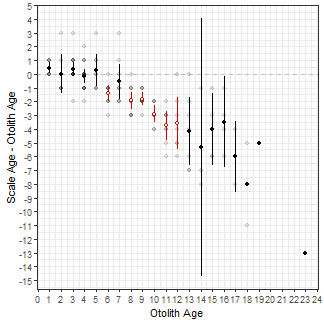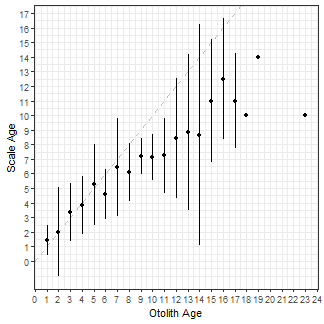Want to share your content on R-bloggers? click here if you have a blog, or here if you don't.

# Guest Post Note

Please note that this is a guest post to `fishR` by Michael Lant, who at the time of this writing is a Senior at Northland College. Thanks, Michael, for the contribution to `fishR`.

# Introduction

My objective is to demonstrate how to create the age bias plots using `ggplot2` rather than functions in `FSA`. Graphs produced in `ggplot2` are more flexible than plots from `plot()` and `plotAB()` in the `FSA` package. Below I will show how to use `ggplot2` to recreate many of the plots shown in the examples for `plot()` and `plotAB()` in `FSA`.

The code in this post requires functions from the `FSA`, `ggplot2`, and `dplyr` packages.

For simplicity I set `theme_bw()` as the default theme for all plots below. Of course, other themes, including those that you develop, could be used instead.

# The Data

I will use the `WhitefishLC` data from `FSA`. This data.frame contains age readings made by two readers on scales, fin rays, and otoliths, along with consensus readings for each structure.

Additionally, I leverage the results returned by `ageBias()` from `FSA`. As described in the documentation, this function computes intermediate and summary statistics for the comparison of paired ages; e.g., between consensus scale and otolith ages below.

The results of `ageBias()` should be saved to an object. This object has a variety of “data” and “results” in it. For example, the `\$data` object in `ab1` contains the original paired age estimates, the differences between those two estimates, and the mean of those two estimates.

In addition, the `\$bias` object of `ab1` contains summary statistics of ages for the first structure given in the `ageBias()` formula by each age of the second structure given in that formula. For example, the first row below gives the number, minimum, maximum, mean, and standard error of the scales ages that were paired with an otolith age of 1. In addition, there is a t-test, adjusted p-value, and a significance statement for testing whether the mean scale age is different from the otolith age. Finally, confidence intervals (defaults to 95%) for the mean scale age at an otolith age of 1 is given, with a statement about whether a confidence interval could be calculated (see the documentation for `ageBias()` for the criterion used to decide if the confidence interval can be calculated).

The results in `\$bias.diff` are similar to those for `\$bias` except that the difference in age between the two structures is summarized for each otolith age.

These different data.frames will be used in the `ggplot2` code below when creating the various versions of the age-bias plots. Note that at times multiple data frames will be used in the same code so that layers can have different variables.

# Basic Age-Bias Plot

Below is the default age-bias plot created by `plotAB()` in `FSA`.The `ggplot2` code below largely recreates this plot.The specifics of the code above is described below.

• The base data used in this plot is the `\$bias` data.frame discussed above.
• I begin by creating the 45^o^ agreement line (i.e., slope of 1 and intercept of 0) with `geom_abline()`, using a dashed `linetype=` and a gray `color=`. This “layer” is first so that it sits behind the other results.
• I then add the error bars using `geom_errorbar()`. The `aes()`thetics here will map the consensus otolith age to the `x=` axis and the lower and upper confidence interval values for the mean consensus scale age at each consensus otolith age to `ymin=` and `ymax=`. The `color=` of the lines are mapped to the `sig` variable so that points that are significantly different from the 45^o^ agreement line will have a different color (with `scale_color_manual()` described below). Finally, `width=0` assures that the error bars will not have “end caps.”
• Points at the mean consensus scale age (`y=`) for each otolith age (`x=`) are then added with `geom_point()`. Again, the `color=` and `fill=` are mapped to the `sig` variable so that they will appear different depending on whether the points are significantly different from the 45^o^ agreement line or not. Finally, `shape=21` represents a point that is an open circle that is outlined with the `color=` color and is filled with the `fill=` color.
• `scale_fill_manual()` and `scale_color_manual()` are used to set the colors and fills for the levels in the `sig` variable. Note that `guide="none"` is used so that a legend is not constructed for the colors and fills.
• `scale_x_continuous()` and `scale_y_continuous()` are used to set the labels (with `name=`) and axis breaks for the x- and y-axes, respectively. The names are drawn from labels that were given in the original call to `ageBias()` and stored in `ab1`.

The gridlines and the size of the fonts could be adjusted by modifying theme, which I did not do here for simplicity.

# More Examples

Below are more examples of how `ggplot2` can be used to recreate graphs from `plot()` in `FSA`. For example, the following plot is very similar to that above, but uses the `\$bias.diff` object in `ab1` to plot mean differences between scale and otolith ages against otolith ages. The reference for the differences is a horizontal line at 0 so `geom_abline()` from above was replaced with `geom_hline()` here.The graph below is similar to above but includes the raw data points from `\$data` and colors the mean (and confidence intervals) for the differences based on the significance as in the first plot. Because data were drawn from different data frames (i.e., `ab1\$data` and `ab1\$bias.diff`) the `data=` and `mapping=` arguments had to be moved into the specific `geom_`s. Note that the raw data were made semi-transparent to emphasize the over-plotting of the discrete ages.The graph below is the same as above except that a loess smoother has been added with `geom_smooth()` to emphasize the trend in the differences in ages. The smoother should be fit to the raw data so you must be sure to use `ab1\$data`. I left the default blue color for the smoother and changed the width of the default line slightly by using `size=.65`.# What Prompted This Exploration

Graphics made in `ggplot2` are more flexible than the ones produced in `FSA`. For example, we recently had a user ask if it was possible to make an “age-bias plot” that used “error bars” based on the standard deviation rather than the standard error. While it is questionable whether this is what should be plotted it is nevertheless up to the user and their use case. Because this cannot be done using the plots in `FSA` we turned to `ggplot` to make such a graph.

Standard deviation was not returned in any of the `ageBias()` results (saved in `ab1`). However, the standard error and sample size were returned in the `\$bias` data frame. The standard deviation can be “back-calculated” from these two values using `SD=SE*sqrt(n)`. I then created two new variables called `LSD` and `USD` that are the means minus and plus two standard deviations. All three of these variables are added to the `\$bias` data.frame using `mutate()` from the `dplyr` package.

A plot like the very first plot above but using two standard deviations for the error bars is then created by mapping `ymin=` and `ymax=` to `LSD` and `USD`, respectively, in `geom_errorbar()`. Note that I removed the color related to the significance test as those don’t pertain to the results when using the standard deviations to represent “error bars.”Finally, to demonstrate the flexibility of using `ggplot` with these type of data, I used a violin plot to show the distribution of scale ages for each otolith age while also highlighting the mean scale age for each otolith age. The violin plots are created with `geom_violin()` using the raw data stored in `\$data`. The `group=` must be set to the x-axis variable (i.e., otolith age) so that a separate violin will be constructed for each age on the x-axis. I `fill`ed the violins with `grey` to make them stand out more.